Question

# A 500 kg elevator accelerates upward at 2 m/s2 for 17 m, starting from rest. A)...

A 500 kg elevator accelerates upward at 2 m/s2 for 17 m, starting from rest.

A) How much work does gravity do on the elevator?

B) How much work does the tension in the elevator cable do on the elevator?

C) What is the elevator’s kinetic energy after traveling 17 m?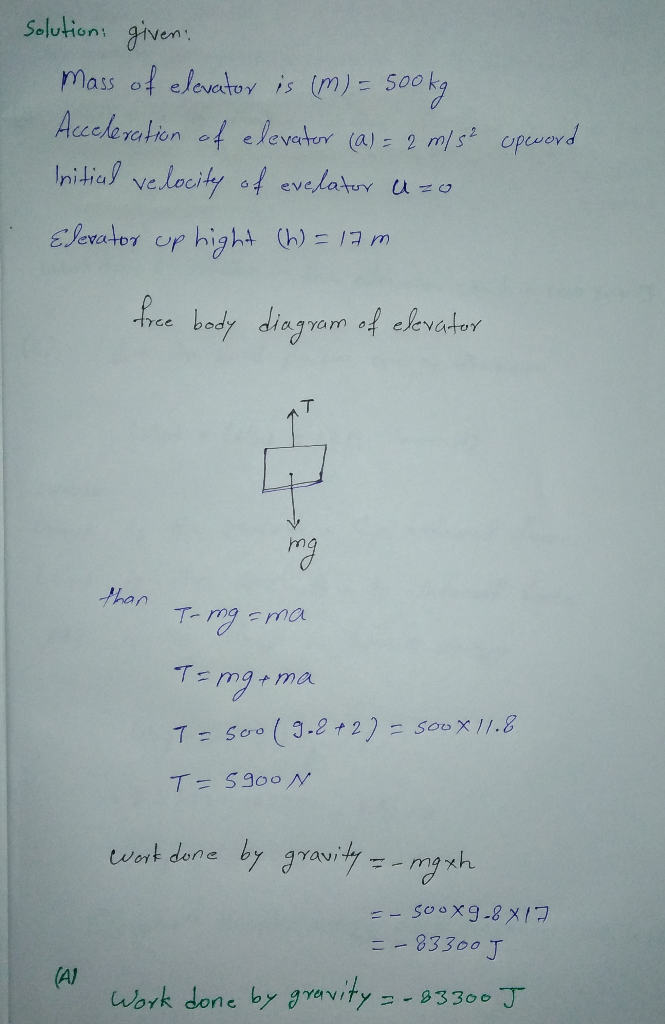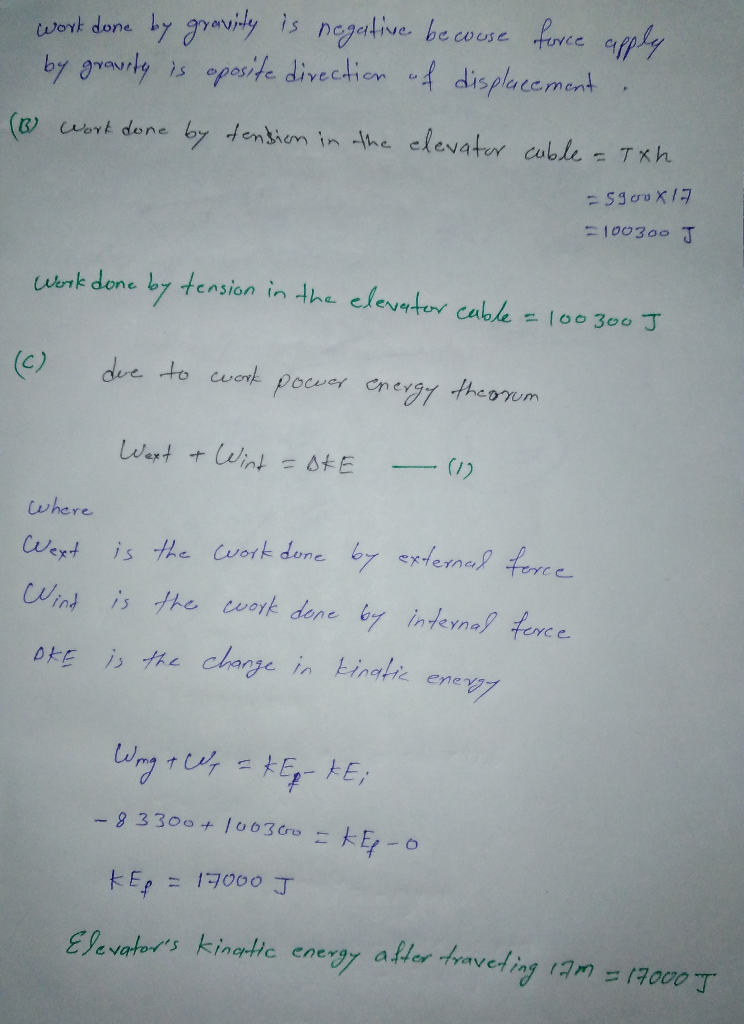#### Earn Coins

Coins can be redeemed for fabulous gifts.

Similar Homework Help Questions
• ### A 900 kg elevator accelerates upward at 1.3 m/s2 for 11 m, starting from rest.

A 900 kg elevator accelerates upward at 1.3 m/s2 for 11 m, starting from rest. You may need to review (Page 206) Part A How much work does gravity do on the elevator?Part B How much work does the tension in the elevator cable do on the elevator? Part C What is the elevator's kinetic energy after traveling 11 m?

• ### 5. An elevator filled with passengers has a mass of 1570 kg a.) The elevator accelerates...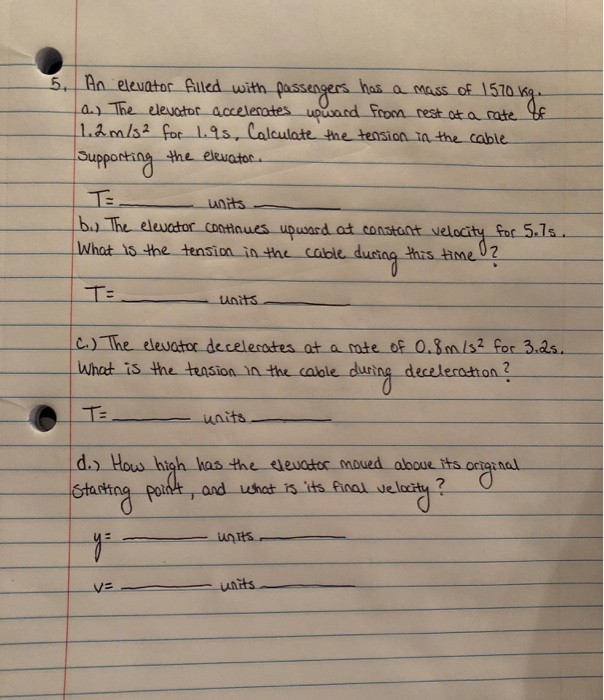5. An elevator filled with passengers has a mass of 1570 kg a.) The elevator accelerates upward from rest at a rate of 11.2 m/s² for 1.95, Calculate the tension in the cable Supporting the elevator. b.) The elevator continues upward at constant velocity for 5.ls. What is the tension in the cable during this time? TE_ Units c.) The elevator decelerates at a rate of 0.8m/s² for 3.2s. What is the tension in the cable during deceleration ? T=...

• ### A 2055 kg elevator accelerates upward at 1.500 m/s? What is the tension in the cable...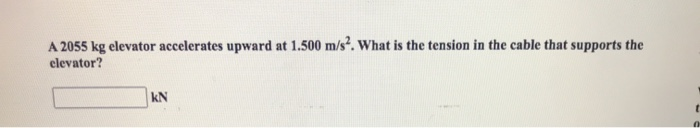A 2055 kg elevator accelerates upward at 1.500 m/s? What is the tension in the cable that supports the elevator? KN 0

• ### 018 (part 1 of 2) 10.0 points An elevator accelerates upward at 1.2 m/s2. The acceleration...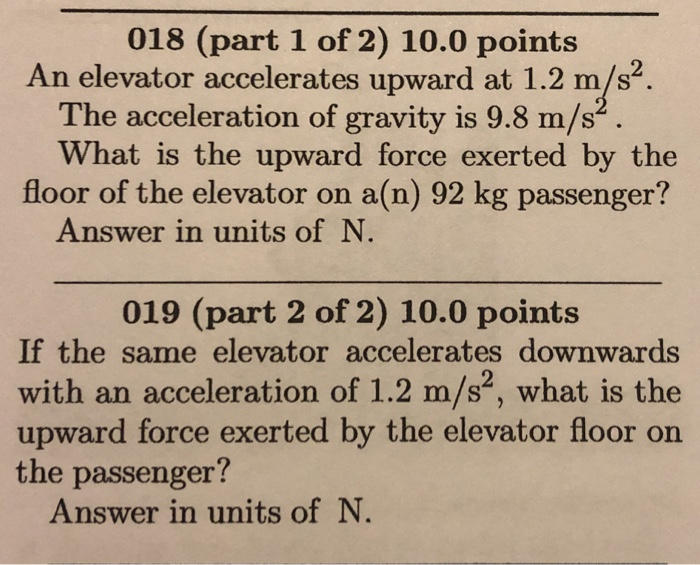018 (part 1 of 2) 10.0 points An elevator accelerates upward at 1.2 m/s2. The acceleration of gravity is 9.8 m/s What is the upward force exerted by the floor of the elevator on a(n) 92 kg passenger? Answer in units of N 019 (part 2 of 2) 10.0 points If the same elevator accelerates downwards with an acceleration of 1.2 m/s, what is the upward force exerted by the elevator floor on the passenger? Answer in units of

• ### Two blocks of mass m = 13.3 kg each are fastened to the ceiling of an elevator. The elevator accelerates upward at a =...

Two blocks of mass m = 13.3 kg each are fastened to the ceiling of an elevator. The elevator accelerates upward at a = 1.51 m/s2. Find the tension in the bottom rope? Find the tension in the top rope.

• ### Two blocks of mass m = 14.7 kg each are fastened to the ceiling of an elevator..The elevator accelerates upward at a =...

Two blocks of mass m = 14.7 kg each are fastened to the ceiling of an elevator..The elevator accelerates upward at a = 1.62 m/s2. Find the tension in the bottom rope. Find the tension in the top rope.

• ### Two blocks of mass m = 10.8 kg each are fastened to the ceiling of an elevator. The elevator accelerates upward at a...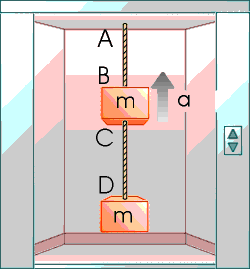Two blocks of mass m = 10.8 kg each are fastened to the ceiling of an elevator. The elevator accelerates upward at a = 2.00 m/s2. Find the tension in the bottom rope and the top rope.

• ### Two blocks are fastened to the ceiling of an elevator. The elevator accelerates upward at 1.50 m/s2. The blocks both ha...

Two blocks are fastened to the ceiling of an elevator. The elevator accelerates upward at 1.50 m/s2. The blocks both have a mass of 12.5 kg. Find the tension in each rope. top rope N bottom rope N

• ### on a (a) The elevator accelerates upward from rest at a rate of 1.25 m/s? for...on a (a) The elevator accelerates upward from rest at a rate of 1.25 m/s? for 1.50 s. What does the scale read during this 1.50s interval? (b) The elevator continues upward at constant velocity for 8.50 s, what does the scale read nos? (c) While still moving upward, the elevator's speed decreases at a rate of 0.700 m/s? for 3.00 s. What is the scale reading during this time? Tutorial In an exciting game, a baseball player manages to...

• ### An elevator car with a mass of equal to 800 kg is pulled upward at constant...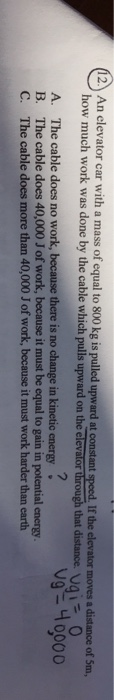An elevator car with a mass of equal to 800 kg is pulled upward at constant speed. If the elevator moves a distance of 5m, how much work was done by the cable which pulls upward on the elevalor through that distance. 12 vg40000 The cable does no work, because there is no change in kinetic energy B. The cable does 40,000 J of work, because it must be equal to gain in potential energy The cable does more than...